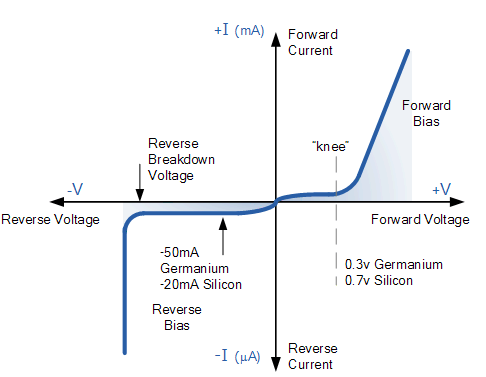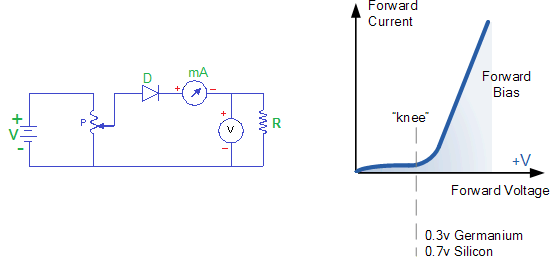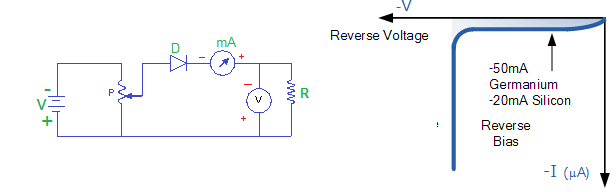# Characteristics of P-N Diode

The variation of current through a diode with applied voltage represented in graph by a curve are called characteristics of P-N Junction diode. It is just a simple graph.Characteristics of P-N Diode

Characteristics of P-N Diode are of two types:

1. Forward Bias Characteristics: It is the graph showing the variation of current with forward biasing voltage as shown in figure.Circuit diagram and graph of forward biased characteristics

The figure shows circuit arrangement for obtaining the forward characteristics of diode. The milli-ammeter (mA) measures the current while the voltmeter V measures potential difference. The forward voltage is gradually increased and the corresponding milli-ammeter reading is noted. The graph is shown in figure c. No current flows until it crosses barrier voltage and as it overcomes the barrier voltage, the current increases rapidly. The forward voltage beyond which the diode current increases rapidly is known as knee voltage. It is 0.3 V for germanium and 0.7 V for silicon.

2. Reverse-bias characteristics: It is the graph showing the variation of circuit current with reverse biasing voltage as shown in figure.Circuit diagram and graph of reverse biased characteristics

The figure shows the circuit arrangement for obtaining the reverse characteristic of a diode. Here the micro ammeter μA. measures reverse current while the voltmeter measures the biasing voltage. The reverse biasing voltage is gradually increased and the corresponding micro ammeter reading is noted. In reverse bias, the reverse current is found to be very small over a long range and it slightly increases with the increase in bias voltage as shown in figure below. When the reverse bias voltage is gradually increased, at one point the current suddenly rises. The voltage where reverse current increases sharply is known as reverse breakdown voltage.

Do you like this article ? If yes then like otherwise dislike : 1